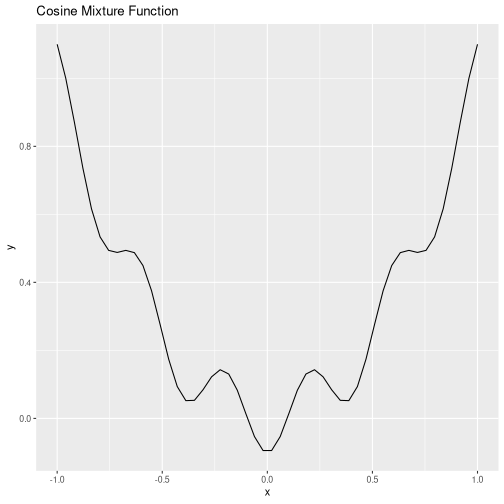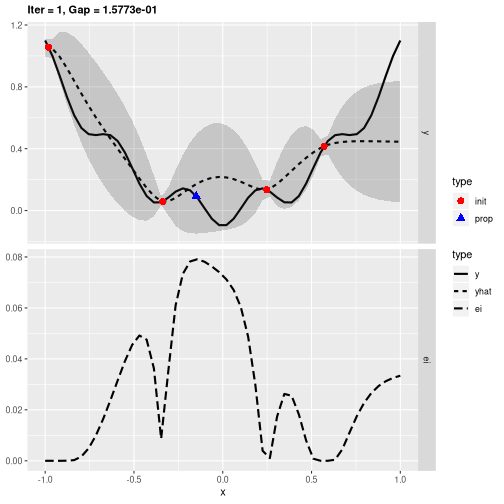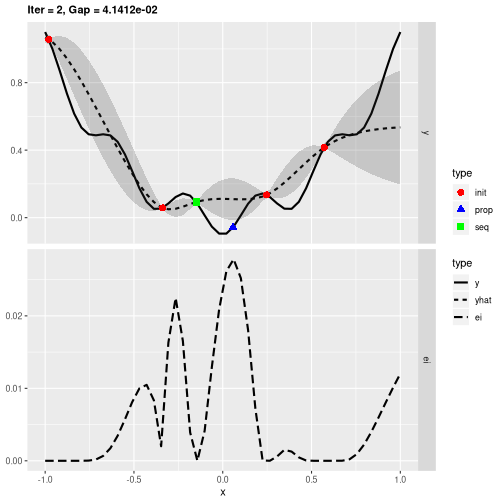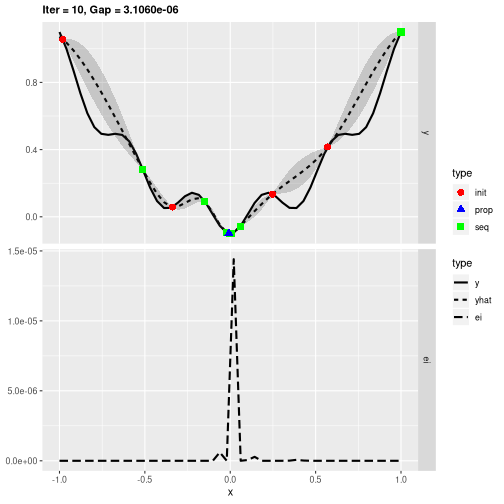# Overview

The main goal of `mlrMBO` is to optimize expensive black-box functions by model-based optimization (aka Bayesian optimization) and to provide a unified interface for different optimization tasks and algorithmic MBO variants. Supported are, among other things:

• Efficient global optimization (EGO) of problems with numerical domain and Kriging as surrogate
• Using arbitrary regression models from mlr as surrogates
• Built-in parallelization using multi-point proposals
• Mixed-space optimization with categorical and subordinate parameters, for parameter configuration and tuning
• Multi-criteria optimization

This vignette gives a brief overview of the features of `mlrMBO`. A more detailed documentation can be found on: http://mlr-org.github.io/mlrMBO/.

# Quickstart

## Prerequisites

Installing `mlrMBO` will also install and load the dependencies `mlr`, `ParamHelpers`, and `smoof`. For this tutorial, you also need the additional packages `DiceKriging` and `randomForest`.

``````library(mlrMBO)
``````

## General MBO workflow

1. Define objective function and its parameters using the package `smoof`.
2. Generate initial design (optional).
3. Define `mlr` learner for surrogate model (optional).
4. Set up a MBO control object.
5. Start the optimization with `mbo()`.

As a simple example we minimize a cosine-like function with an initial design of 5 points and 10 sequential MBO iterations. Thus, the optimizer is allowed 15 evaluations of the objective function in total to approximate the optimum.

### Objective Function

Instead of manually defining the objective, we use the smoof package which offers many toy and benchmark functions for optimization.

``````obj.fun = makeCosineMixtureFunction(1)
obj.fun = convertToMinimization(obj.fun)
print(obj.fun)
## Single-objective function
## Name: Cosine Mixture Function
## Description: no description
## Tags: single-objective, discontinuous, non-differentiable, separable, scalable, multimodal
## Noisy: FALSE
## Minimize: TRUE
## Constraints: TRUE
## Number of parameters: 1
##            Type len Def  Constr Req Tunable Trafo
## x numericvector   1   - -1 to 1   -    TRUE     -
## Global optimum objective value of -0.1000 at
##   x
## 1 0
ggplot2::autoplot(obj.fun)
``````You are not limited to these test functions but can define arbitrary objective functions with smoof.

``````makeSingleObjectiveFunction(
name = "my_sphere",
fn = function(x) {
sum(x*x) + 7
},
par.set = makeParamSet(
makeNumericVectorParam("x", len = 2L, lower = -5, upper = 5)
),
minimize = TRUE
)
## Single-objective function
## Name: my_sphere
## Description: no description
## Tags:
## Noisy: FALSE
## Minimize: TRUE
## Constraints: TRUE
## Number of parameters: 2
##            Type len Def  Constr Req Tunable Trafo
## x numericvector   2   - -5 to 5   -    TRUE     -
``````

Check `?smoof::makeSingleObjectiveFunction` for further details.

### Initial Design

Before MBO can really start, it needs a set of already evaluated points - the inital design, as we have to initially learn our first machine learning regression model to propose new points for evaluation. If no design is given (i.e. `design = NULL`), `mbo()` will use a Maximin Latin Hypercube `lhs::maximinLHS()` design with `n = 4 * getNumberOfParameters(obj.fun)` points. If the design does not include function outcomes `mbo()` will evaluate the design first before starting with the MBO algorithm. In this example we generate our own design.

``````des = generateDesign(n = 5, par.set = getParamSet(obj.fun), fun = lhs::randomLHS)
``````

We will also precalculate the results:

``````des\$y = apply(des, 1, obj.fun)
``````

Note: mlrMBO uses `y` as a default name for the outcome of the objective function. This can be changed in the control object.

### Surrogate Model

We decide to use Kriging as our surrogate model because it has proven to be quite effective for numerical domains. The surrogate must be specified as a mlr regression learner:

``````surr.km = makeLearner("regr.km", predict.type = "se", covtype = "matern3_2", control = list(trace = FALSE))
``````

Note: If no surrogate learner is defined, `mbo()` automatically uses Kriging for a numerical domain, otherwise random forest regression.

### MBOControl

The `MBOControl` object allows customization of the optimization run and algorithmic behavior of MBO. It is created with `makeMBOControl()`, and can be modified with further setter-functions.

For further customization there are the following functions:

• `setMBOControlTermination()`: It is obligatory to define a termination criterion like the number of MBO iterations.
• `setMBOControlInfill()`: It is recommended to set the infill criterion. For learners that support `predict.type = "se"` the Confidence Bound `"cb"` and the Expected Improvement `"ei"` are a good choice.
• `setMBOControlMultiPoint()`: Needed, in case you want to evaluate more then just one point per MBO-Iteration you can control this process here. This makes sense for parallelization.
• `setMBOControlMultiObj()`: Needed, in case you want to optimize a multi-objective target function.
``````control = makeMBOControl()
control = setMBOControlTermination(control, iters = 10)
control = setMBOControlInfill(control, crit = makeMBOInfillCritEI())
``````

### Start the optimization

Finally, we start the optimization process and print the result object. It contains the best best found solution and its corresponding objective value.

``````run = mbo(obj.fun, design = des, learner = surr.km, control = control, show.info = TRUE)
## [mbo] 1: x=0.142 : y = 0.0821 : 0.0 secs : infill_ei
## [mbo] 2: x=-0.0588 : y = -0.0568 : 0.0 secs : infill_ei
## [mbo] 3: x=-0.00787 : y = -0.0992 : 0.0 secs : infill_ei
## [mbo] 4: x=0.000914 : y = -0.1 : 0.0 secs : infill_ei
## [mbo] 5: x=-0.00241 : y = -0.0999 : 0.0 secs : infill_ei
## [mbo] 6: x=0.00421 : y = -0.0998 : 0.0 secs : infill_ei
## [mbo] 7: x=-0.000487 : y = -0.1 : 0.0 secs : infill_ei
## [mbo] 8: x=-0.501 : y = 0.253 : 0.0 secs : infill_ei
## [mbo] 9: x=0.0328 : y = -0.086 : 0.0 secs : infill_ei
## [mbo] 10: x=0.000168 : y = -0.1 : 0.0 secs : infill_ei
print(run)
## Recommended parameters:
## x=0.000168
## Objective: y = -0.100
##
## Optimization path
## 5 + 10 entries in total, displaying last 10 (or less):
##                x           y dob eol error.message exec.time            ei
## 6   0.1424200502  0.08209419   1  NA          <NA>     0.001 -3.210524e-02
## 7  -0.0588246736 -0.05680169   2  NA          <NA>     0.000 -2.895199e-02
## 8  -0.0078732127 -0.09917425   3  NA          <NA>     0.000 -4.124451e-03
## 9   0.0009141747 -0.09998885   4  NA          <NA>     0.001 -1.466116e-03
## 10 -0.0024097515 -0.09992256   5  NA          <NA>     0.000 -1.604071e-04
## 11  0.0042120161 -0.09976347   6  NA          <NA>     0.000 -7.865440e-05
## 12 -0.0004870891 -0.09999684   7  NA          <NA>     0.000 -3.759330e-05
## 13 -0.5011545567  0.25296936   8  NA          <NA>     0.000 -1.285448e-05
## 14  0.0327576367 -0.08597808   9  NA          <NA>     0.000 -1.576849e-05
## 15  0.0001680801 -0.09999962  10  NA          <NA>     0.000 -1.056037e-05
##    error.model train.time prop.type propose.time           se        mean
## 6         <NA>      0.026 infill_ei        0.129 6.915038e-02 -0.10692782
## 7         <NA>      0.016 infill_ei        0.134 7.141779e-02 -0.09923456
## 8         <NA>      0.014 infill_ei        0.151 1.174788e-02 -0.09714734
## 9         <NA>      0.015 infill_ei        0.131 1.928583e-03 -0.10030883
## 10        <NA>      0.013 infill_ei        0.136 4.588194e-04 -0.09994165
## 11        <NA>      0.014 infill_ei        0.144 4.002964e-04 -0.09978701
## 12        <NA>      0.014 infill_ei        0.134 8.531091e-05 -0.09999575
## 13        <NA>      0.020 infill_ei        0.136 1.710841e-01  0.48794187
## 14        <NA>      0.022 infill_ei        0.141 6.453531e-03 -0.08427947
## 15        <NA>      0.015 infill_ei        0.142 2.269214e-05 -0.09999971
``````

## Visualization

For more insights into the MBO process, we can also start the previous optimization with the function `exampleRun()` instead of `mbo()`. This augments the results of `mbo()` with additional information for plotting. Here, we plot the optimization state at iterations 1, 2, and 10.

``````run = exampleRun(obj.fun, learner = surr.km, control = control, show.info = FALSE)
``````
``````print(run)
## MBOExampleRun
## Number of parameters        : 1
## Parameter names             : x
## Parameter types             : numericvector
## Global Opt (known)          : -1.0000e-01
## Gap for best point          : 3.1060e-06
## True points per dim.        : 50
## Objectives                    : 1
## Points proposed per iter      : 1
##
## Infill criterion            : Expected improvement (ei)
##   Direction of optimization : maximize
##   Requirement               : SE estimation
##   Components                : se, mean
##   Parameters                : se.threshold=1e-06
## Infill optimizer              : focussearch
## Infill optimizer restarts     : 3
## Final point by                : best.true.y
## Learner                     : regr.km
## Learner settings:
## jitter=FALSE,covtype=matern3_2,control=trace=FALSE
## Recommended parameters:
## x=-0.000483
## Objective: y = -1.000e-01
plotExampleRun(run, iters = c(1L, 2L, 10L), pause = FALSE)